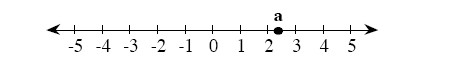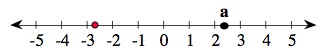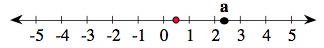### Home > AC > Chapter 1 > Lesson 1.1.4 > Problem1-37

1-37.

Copy the number line below onto your paper. Locate the following numbers by placing the lowercase letters a through e on the number line corresponding to the values given below. Part (a) is done for you.1. $2\ \frac{1}{3}$

1. $−2.7$1. $\frac{1}{2}$1. $−0.2$

Determine on which side of zero the point is located.

1. $33\ \frac{1}{3}\%$ of $12$

Change the percent to a fraction. Then, multiply the decimal by $12$.